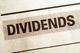# What is dividend yield?

By Research DeskDividend yield is dividend to price ratio. It is the percentage calculated by dividing dividend per share by price per share. Dividend yield is used to calculate the earning on investment (shares) considering only the returns in the form of total dividends declared by the company during the year.

Dividend Yield = Interim + Final Dividend  X 100

Market Price of the share

E.g.: For a company for FY13,

Interim dividend = Rs. 2 per share

Final dividend = Rs. 3 per share

Share price = Rs. 50

Dividend yield = 2 + 3

50

Dividend yield =10%